Hello, readers welcome to new post. Today we will discuss Fsc 1st year physics chapter 10 solved exercise.  In the previous post, we have discuss Fsc 1st year physics chapter 9 solved exercise. Now we will cover chapter 10 with short answers. So let’s get started.

### Fsc 1st year physics chapter 10 solved exercise

Q 10.1

• The size of the image to the size of the object is called linear magnification.
• Its equation is
• M=I/O= q
• The ratio between the angle created by the image seen through optical instruments and the angle of the object seen with a normal eye is called angular magnification.
• M=B/α
• There is no unit for linear and angular magnification
• Convex lense having a short focal length can be used as a magnifying glass when the object is put between the lens and focus the image created is erect and virtual and magnified

Q10. 2

• Angular magnification means how large and magnified the image is created through instruments and resolving power is its ability to give the minor details of objects under observation. Defects that occur in instruments can limit the magnification.

Q 10. 3

Q10.4

• It is caused by a lens defect called chromatic aberration. it is caused of a prism-like creation of a lense in which all rays of white light can not joint at a common point in result image is not sharp and consists of colored edges.

Q10.5

• a: Biconvex lens ray diagram used as a magnifying glass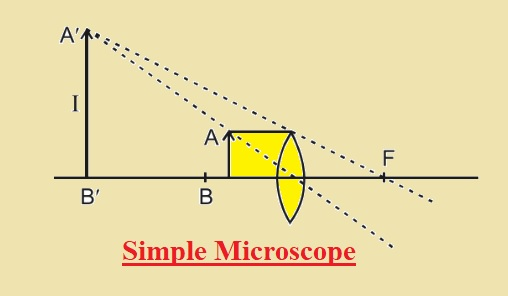• b: Ray diagram of two convex lenses used to make a compound microscope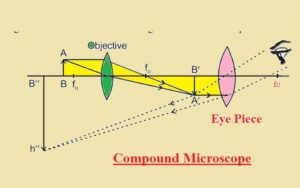Q10. 6

• The person will observe the complete image of the moon if half of the objective lens of the telescope is covered and its brightness decreases since less light is transmitted in the lens

Q10. 7

• As we given
• M= 5
• Large Distance =q=  25cm
• lens focal lenght= f=?
• As we know that
• M=1+d/f
• 5=1+25/f
• f=6.2cm

Q10. 8

• 1. The formula for resolving the power of a lens having Dia D is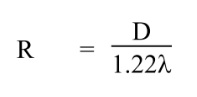• From the equation resolving power depends on the dia so B  is answer
• ii. As we know the resolving power of an astronomical telescope depends on the dia of the objective lens so is correct

Q 10.9

• It consists of a thin core with a diameter of five micrometers and a large size of cladding. It has the ability to handle more than fourteen tv channels or fourteen thousand phone calls so used in telecommunications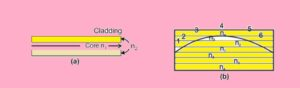Q 10.10

• Two principle uses for transmission through optical fiber
• Total internal reflection
• Continous refraction

Q 11.11

• If a light source is not monochromatic light will disperse when moving through core of optical fiber into different wavelengths Light of different wavelengths takes different times to reach the second point of fiber hence signal is distorted power losses due to dispersion in the fiber.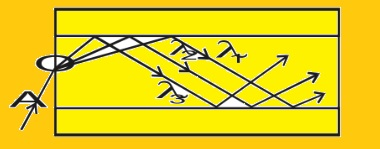That is all about the Fsc 1st year physics chapter 10 solved exercise all details has explained. IF you have any further question ask in the comments.